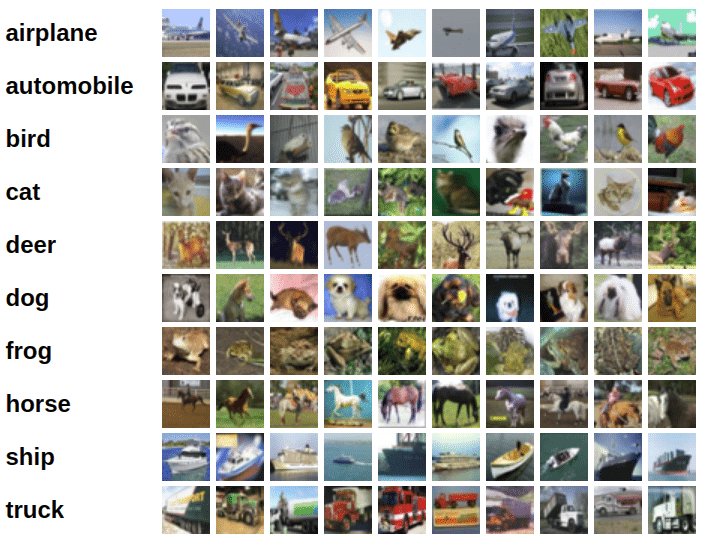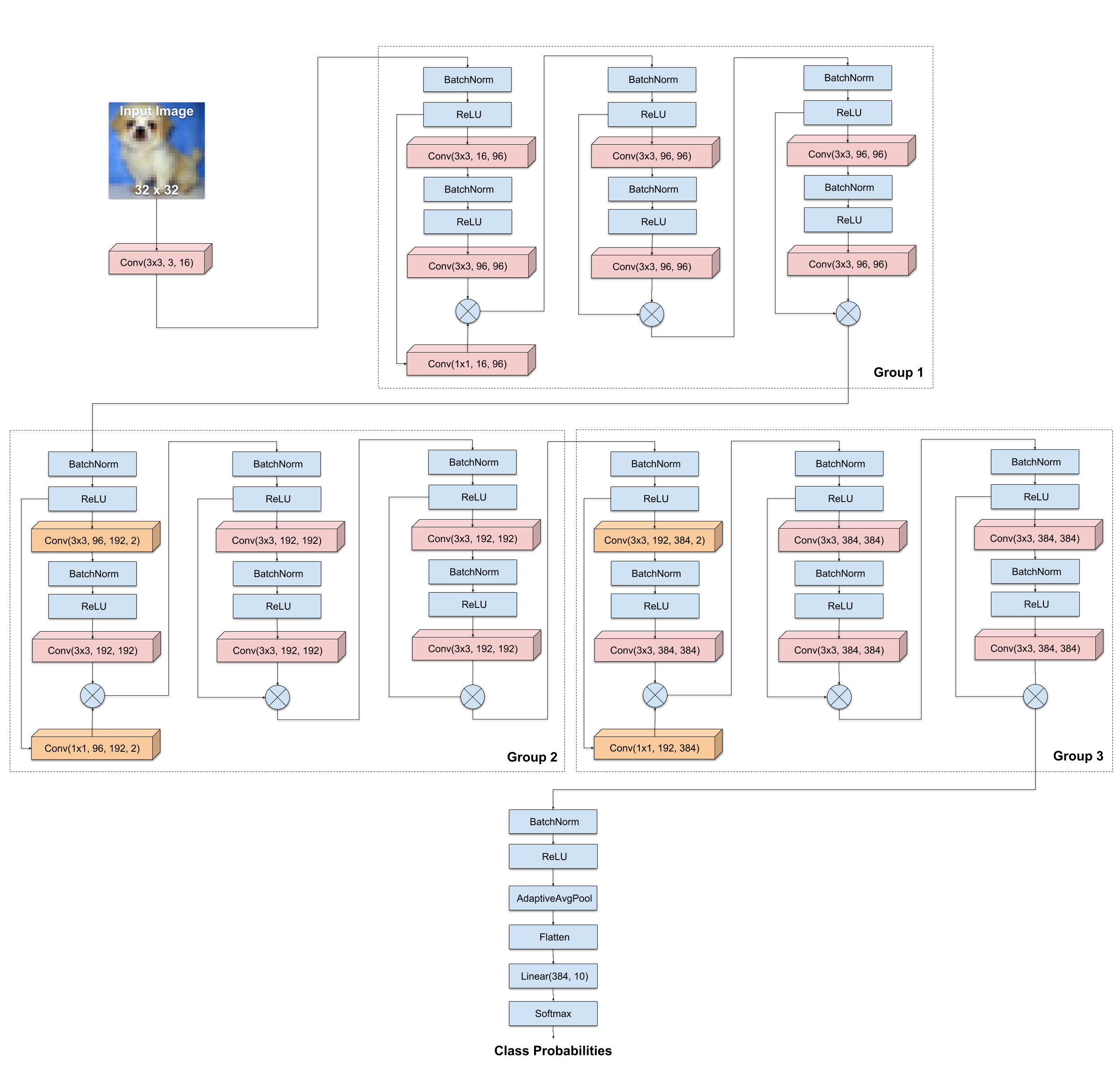# Implement ResNet using PyTorch

4 minute read

This note book presents how to build a ResNet using PyTorch

# CIFAR-10 Dataset

The CIFAR-10 dataset consists of 60k 32x32 color images in 10 classes, with 6k images per class. There are 50k training images (5k per class) and 10k test images (1k per class). Here are 10 random images from each class:Image Source: Convolutional Neural Networks

I worked locally at first. To download CIFAR-10 dataset, I run the following commands (Mac OS):

``````mkdir data
cd data
curl -O http://files.fast.ai/data/cifar10.tgz
tar -xf cifar10.tgz
``````

Also, you can use PyTorch buildin function torchvision.datasets.CIFAR10() to download the image, as I did in CovNet-PyTorch.

# Python Library Needed

All the Python library needed in this program is listed below as an overview. In implementation, the libraries are loaded when needed.

``````# util libraries
import os
``````
``````# Scientific computation
import numpy as np
``````
``````# NN libraries
import torch
import torchvision
import torchvision.transforms as transforms
from torchvision.datasets import ImageFolder
#from torch.utils.data import DataLoader  # somehow does not work
``````
``````# fastai
#from fastai.dataset import ModelData
#ModuleNotFoundError: No module named 'fastai.dataset'
``````

# Define ConvNet

In the following scripts, CovNet2L is a simple 2 layer ConvNet class that inherits from the master torch.nn.Module class. It is the same as that is defined in ConvNet-PyTorch.ipynb.

ConNet2L only have layers CONV-MAXPOOL-FC-FC.

``````from torch.autograd import Variable
import torch.nn.functional as nnFunc

class ConvNet2L(torch.nn.Module):

# To do later maybe -- change to accept as input kernel_size, stride, padding
def __init__(self):
super(ConvNet2L, self).__init__()

# Input channels=3, output channels = 18
self.conv1 = torch.nn.Conv2d(3, 18, kernel_size=3, stride=1, padding=1)
self.pool = torch.nn.MaxPool2d(kernel_size=2, stride=2, padding=0)

# 18 x 16 x 16 input features, 64 output features
self.fc1 = torch.nn.Linear(18*16*16, 64)

# 64 input feature, 10 output for 10 defined classes
self.fc2 = torch.nn.Linear(64, 10)

def forward(self, x):

# CONV Layer
# Size changes from raw image (3, 32, 32) to (18, 32, 32)
x = nnFunc.relu(self.conv1(x))

# POOL Layer
x = self.pool(x)

# Reshape data to vector to the FC layer
# Size changes from (18, 16, 16) to (1, 18*16*16)
x = x.view(-1, 18*16*16)

# FC Layer
# Size changes from (1, 18*16*16) to (1, 64)
x = nnFunc.relu(self.fc1(x))

# FC Layer
# Size changes from (1, 64) to (1, 10)
x = self.fc2(x)

return(x)
``````

# Define ResNet Architecture

I will contruct a ResNet Architecture called WideResNet-22. It is inspired from the family of architectures introduced in the paper Wide Residual Networks. It has the following architecture:Image Source: Training an Image Classifier from scratch in 15 minutes

# Function to Load Data

``````working_dir = os.getcwd()
data_dir = 'data/cifar10/'  # don't use '/data/cifar10/', no file error
train_dir = data_dir + 'train'
test_dir = data_dir + 'test'
print(working_dir)
print(data_dir)
print(train_dir)
print(test_dir)
``````
``````/Users/elynn/Dropbox/__openAI/openai
data/cifar10/
data/cifar10/train
data/cifar10/test
``````
``````# load image files from disk and do transformation

# define tranformation functions, preproscessing image here
# Normalization + data augmentation
transform = [transforms.ToTensor(), transforms.Normalize((0.5, 0.5, 0.5), (0.5, 0.5, 0.5))]
normalization_transform = transforms.Compose(transform)
augmentation_transform = transforms.Compose([transforms.RandomCrop(32, padding=4), transforms.RandomHorizontalFlip()] + transform)
``````
``````# Load CIFAR-10 using PyTorch buildin function ImageFolder()
# To make things simple, here I use 'cirfa-10/test' as the validation dataset. And use augmented dataset 'aug_ds' for testing. In ConvNet-PyTorch.ipynb, I use random sampler function to divide 'cirfa-10/train' into train and validation sets.

train_ds = ImageFolder(train_dir, augmentation_transform) # type(train_ds); dir(train_ds)
test_ds = ImageFolder(test_dir, normalization_transform)
aug_ds = ImageFolder(test_dir, augmentation_transform)
``````
``````# hyperparameters to be set up

# same batch_size for all training, validation, testing data
batch_size = 32
num_workers = 2  # leverage multi-core CPUs to load the images and apply transformation faster

# Load data # dir(train_loader)
train_loader = torch.utils.data.DataLoader(train_ds, batch_size=batch_size, shuffle=True, num_workers=num_workers, pin_memory=True)
vali_loader = torch.utils.data.DataLoader(test_ds, batch_size=batch_size, shuffle=False, num_workers=num_workers, pin_memory=False)
test_loader = torch.utils.data.DataLoader(aug_ds, batch_size=batch_size, shuffle=False, num_workers=num_workers, pin_memory=True)
``````

# Function to train NN

``````import time
from torch.autograd import Variable
import torch.optim as optim

# train NN

# need to define net has parameter
# INPUT: net, batch_size, n_epochs, learning_rate
learning_rate = 0.001

def trainNN(net, batch_size, n_epochs, learning_rate, train_loader, vali_loader):

# Print all the hyperparameters of the training iteration:
print("####### HYPERPARAMETERS #######")
print("batch_size = ", batch_size)
print("epochs = ", n_epochs)
print("learning_rate = ", learning_rate)
print("#"*31)

n_batches = len(train_loader)

# Create loss function
loss = torch.nn.CrossEntropyLoss()

# Optimizer
optimizer = optim.Adam(net.parameters(), lr=learning_rate)

# Training start time
training_start_time = time.time()

for epoch in range(n_epochs):

print("Epoch = ", epoch+1)
running_loss = 0.0
print_every = n_batches // 10
start_time = time.time()
total_train_loss = 0

# in each epoch do ...
# epoch = 1
for i, data in enumerate(train_loader):

# Get data = inputs, labels one batch at a time
inputs, labels = data

# Wrap them in a Variable object
inputs, labels = Variable(inputs), Variable(labels)

# Set the parameter gradients to zero
optimizer.zero_grad()

# Forward pass, backward pass, optimize
outputs = net(inputs)
loss_size = loss(outputs, labels)
loss_size.backward()
optimizer.step()

# Print statistics
running_loss += loss_size.item()
total_train_loss += loss_size.item()

# Print every 10-th batch of a epoch
if (i+1)%(print_every+1)==0:
print("Epoch {}, {:d}% \t train_loss: {:.2f} took: {:.2f}s".format(epoch+1, int(100 * (i+1) / n_batches), running_loss / print_every, time.time() - start_time))
# Reset running loss and time
running_loss = 0.0
start_time = time.time()

# At the end of the epoch, do a pass on the validation set
total_vali_loss = 0
for inputs, labels in vali_loader:
# Wrap tensors in Variables
inputs, labels = Variable(inputs), Variable(labels)

# Forward pass
vali_outputs = net(inputs)
vali_loss_size = loss(vali_outputs, labels)
total_vali_loss += vali_loss_size.item()

print("Validation loss = {:.2f}".format(total_vali_loss / len(vali_loader)))

print("Training finished, took {:.2f}s".format(time.time() - training_start_time))

``````
``````# data sampler function
# for training, validation and testing.
from torch.utils.data.sampler import SubsetRandomSampler

``````

# Train ConvNet

``````trainNN(ConvNet1, batch_size=32, n_epochs=5, learning_rate=0.001, train_loader=train_loader, vali_loader=vali_loader)
``````

# Train ResNet

``````
``````

Updated: